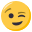# Objectpointer

• Hello,

I am working on the following problem. There are two different classes A and B where each class handles separate objects. A third class C is used as interface with other program parts. Each class is saved in an separate file. For the interaction between the different objects of A and B I need to have a pointer to A inside B.

I already implemented the following code which works great.

File A

``````[cpp]
class C;

class A
{
private:
int x_;
C* const ptrC_;
public:
A(int x, C* const ptrC) : x_(x), ptrC_(ptrC) {}
};
[/cpp]
``````

File B

``````[cpp]
class C;

class B
{
private:
int y_;
C* const ptrC_;
public:
B(int y, C* const ptrC) : y_(y), ptrC_(ptrC) {}
};
[/cpp]
``````

File C

``````[cpp]
#include "A.h"
#include "B.h"

class A;
class B;

class C
{
private:
int z_;
A class_a;
B class_b;
public:
C(int x, int y, int z) : z_(z), class_a(x, this), class_b(y, this) {}
};
[/cpp]
``````

But when I add the reference to A in B the compiler complains that the field class_b has incomplete type.

File B

``````[cpp]
[b]#include "A.h"[/b]

[b]class A;[/b]
class C;

class B
{
private:
int y_;
[b]A* const ptrA_;[/b]
C* const ptrC_;
public:
B(int y, [b]A* const ptrA,[/b] C* const ptrC) : y_(y), [b]ptrA_(ptrA),[/b] ptrC_(ptrC) {}
};
[/cpp]
``````

File C

``````[cpp]
#include "A.h"
#include "B.h"

class A;
class B;

class C
{
private:
int z_;
A class_a;
B class_b;
public:
C(int x, int y, int z) : z_(z), class_a(x, this), class_b(y, [b]&class_a,[/b] this) {}
};
[/cpp]
``````

Can you please help me to figure out what I did wrong.

Thank you very much.

• Your example contains a lot of errors (missing ; at class end, missing "" around included files, use of `C*` in `A` without declaration of `class C;` ). I don't know whether they are related to your problem or not.

Can you show us an code example of the three files which contains only the mentioned issue? Then we don't have to guess.

• Thank you very much for your fast reply and your suggestions. I have corrected the wrong written parts of the code in my case description.

I would only be too pleased to paste the whole code in this forum. The problem is the size of the files. So I had to break down the code to the core of the problem.

• I appreciate that you posted a minimal example. But you should try to keep it minimal and correct (apart from the problem, of course)Assuming the header `"A.h"` is surrounded with include guards, the only mistake I see is the missing declaration of `C` in

``````class A
{
private:
int x_;
C* const ptrC_; // <- use of unknown type C
public:
A(int x, C* const ptrC);
};
``````

You can fix that by inserting the forward declaration

``````class C;
``````

above the class definition of `A` .

• Thank you very much for your help. I took a deeper look into my code after I read your hints and found that I included the header of class C

``````# include "C.h"
``````

in the file of

``````class A
``````

After I deleted the include statement the error was gone.

Thank you.